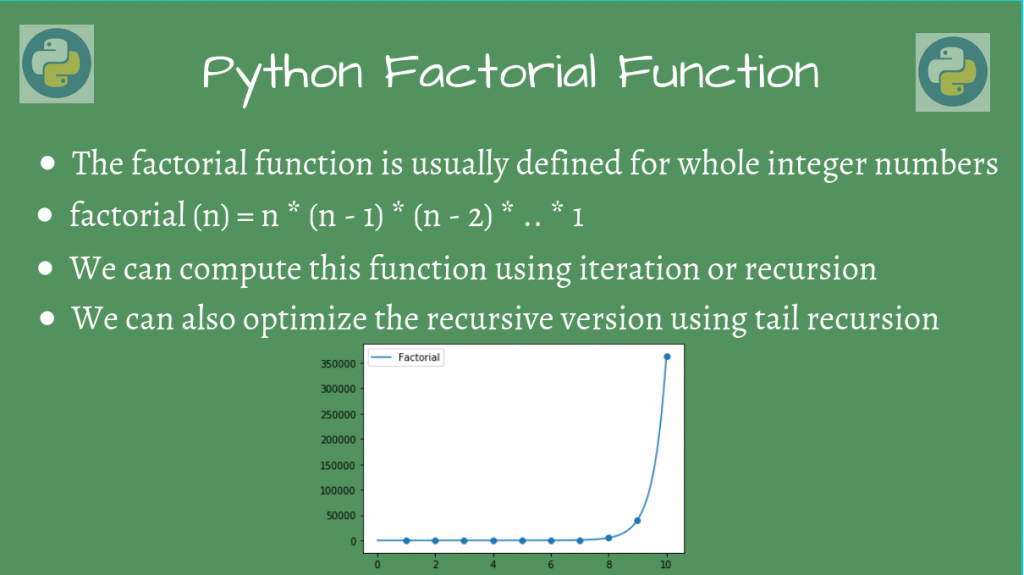# Python Factorial ExamplesIn this article, we’ll look at how we can calculate the Python factorial using different approaches.

## The Python Factorial function

The Python factorial function `factorial(n)` is defined for a whole number `n`. This computes the product of all terms from `n` to `1`. `factorial(0)` is taken to be `1`.

So, the function is:

```factorial(n) = n * (n-1) * (n-2) * ... * 1, n >= 1
factorial(n) = 1, n = 0
```

Therefore, factorial(4) = 4 * 3 * 2 * 1 = 24.

Let’s analyze how we can write this mathematical function in Python.

## Using math.factorial()

We can directly use the `math` module’s factorial function to do the work for using:

```import math

def factorial(x):
return math.factorial(x)

print(factorial(5))
```

Output

```120
```

We’ll also look at finding this function using other methods: let’s use an iterative procedure now.

## Using an Iterative Procedure

We can directly loop over all the numbers for 1 to n and directly multiply the product.

```def factorial(n):
if n == 0:
return 1
prod = 1
for i in range(1, n+1):
prod = prod * i
return prod

if __name__ == '__main__':
print(factorial(4))
print(factorial(7))

```

Output

```24
5040
```

Let’s now look at using a recursive method for the Python factorial function.

## Using a Recursive Procedure

We can utilize recursion, to compute this function. Basically, we reduce this function into a smaller sub-problem. After we compute the sub-problems, we can combine the results to give the final answer.

Since the problem structure is a decreasing product, we can model the recursion in the following manner:

```factorial(n) = n * factorial(n-1), n >= 1
factorial(0) = 1, n = 0
```

The last line is the base case. This is the point where the recursion stops, and we can get the final product, when the recursion unwinds.

We’ll write the corresponding Python function for this:

```def factorial(n):
if n == 0:
# Base case n = 0
return 1
else:
# Use the definition of factorial function
return n * factorial(n-1)

if __name__ == '__main__':
print(factorial(4))
print(factorial(7))
```

Output

```24
5040
```

That seems to be correct. Let’s analyze what actually happens in the recursion calls.

Whenever recursion calls are used, there is a call stack, which continuously stores the state of the program, until the base case is reached. The stack elements are finally popped out one by one after a value is returned by the corresponding block, when the recursion unwinds from `n = 0`.

The whole process is explained in the below figure, for finding `fact(3)`. The first part of the entire process is the build-up of the stack where each of those recursive calls is stacked on top of each other until the function returns 1.

Once the function can no longer recursively call, it starts calculating the factorial as demonstrated below.

When the functions return, the stack elements get popped out one by one, from the top. When it finally reaches the `main()` stack, the function is finally complete, and we have our value, which comes out to be `6`.

## Tail Recursive Calls

While our program works fine, the problem with our recursive function is that the stack size grows as much as the input size.

So if `n` is a very large number, our recursion stack may be very large, that that may cause the stack to overflow! To avoid this, we’ll use another approach to coding a recursive function, called a tail-recursive procedure.

The tail procedure call aims to perform the recursion call after computing the intermediate result. So, instead of increasing the stack size, the program can use the same stack for the whole process! It only needs to be updated.

This means that our recursive call must always be at the end. This is why it is a “tail call”.

```def fact_helper(accum, n):
if n == 0:
return accum
return fact_helper(accum*n, n-1)

def factorial(n):
return fact_helper(1, n)

if __name__ == '__main__':
print(factorial(4))
print(factorial(7))
```

Since we cannot directly make the recursive call at the end, we do it with another helper function, that does that actual computation for us. This helper function stores an `accumulator`, which stores the current value of the function.

The trick is to pass the accumulator as a parameter to the recursive function, and update it, using `accum*n`. This way, we will store the intermediate state in one variable, and hence, only in one stack frame!

Output

```24
5040
```

You get the same output as before! Now, you’ve also ensured that the program uses only one stack frame, so it is essentially equivalent to the iterative procedure! Isn’t that nice?

## Conclusion

In this article, we learned about how we can implement the factorial function in different ways, using the math module, as well as through both iteration and recursion.